Courses

# Legendre Special Function (Part - 6)- Mathematical Methods of Physics, UGC - NET Physics Physics Notes | EduRev

## Physics for IIT JAM, UGC - NET, CSIR NET

Created by: Akhilesh Thakur

## Physics : Legendre Special Function (Part - 6)- Mathematical Methods of Physics, UGC - NET Physics Physics Notes | EduRev

The document Legendre Special Function (Part - 6)- Mathematical Methods of Physics, UGC - NET Physics Physics Notes | EduRev is a part of the Physics Course Physics for IIT JAM, UGC - NET, CSIR NET.
All you need of Physics at this link: Physics

Polar Angle Dependence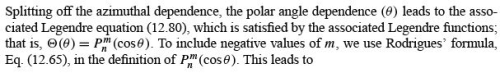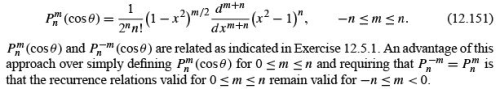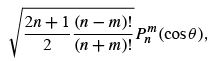−n ≤ m ≤ n,              (12.152)

which are orthonormal with respect to the polar angle θ .

Spherical Harmonics

The function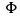m (ϕ ) (Eq. (12.150)) is orthonormal with respect to the azimuthal angle ϕ . We take the product ofm (ϕ ) and the orthonormal function in polar angle from Eq. (12.152) and deﬁne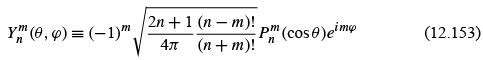to obtain functions of two angles (and two indices) that are orthonormal over the spherical surface. These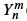(θ , ϕ ) are spherical harmonics, of which the ﬁrst few are plotted in Fig. 12.15. The complete orthogonality integral becomes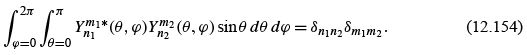The extra (−1)m included in the deﬁning equation of(θ , ϕ ) deserves some comment.
It is clearly legitimate, since Eq. (12.144) is linear and homogeneous. It is not necessary, but in moving on to certain quantum mechanical calculations, particularly in the quantum theory of angular momentum (Section 12.7), it is most convenient. The factor (−1)m is a phase factor, often called the Condon–Shortley phase, after the authors of a classic text on atomic spectroscopy. The effect of this (−1)m (Eq. (12.153)) and the (−1)m of Eq. (12.73c) for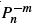(cos θ) is to introduce an alternation of sign among the positive m spherical harmonics. This is shown in Table 12.3.
The functions(θ , ϕ ) acquired the name spherical harmonics ﬁrst because they are deﬁned over the surface of a sphere with θ the polar angle and ϕ the azimuth. The harmonic was included because solutions of Laplace’s equation were called harmonic functions and(cos,ϕ ) is the angular part of such a solution.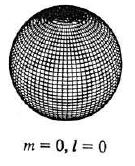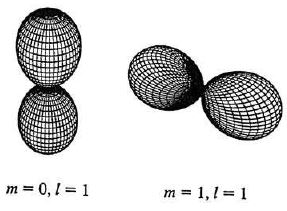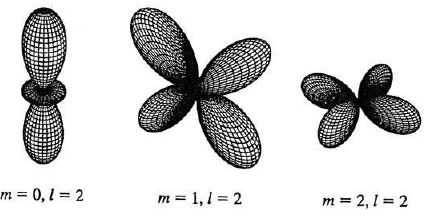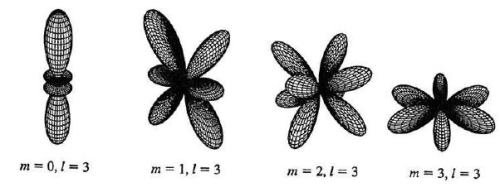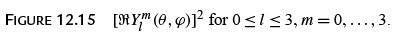Table 12.3 Spherical Harmonics (Condon–Shortley Phase)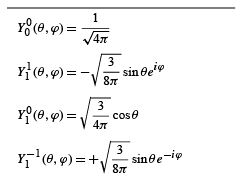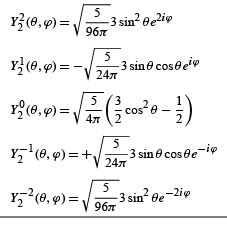In the framework of quantum mechanics Eq. (12.145) becomes an orbital angular momentum equation and the solution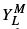(θ , ϕ ) (n replaced by L, m replaced by M )isan angular momentum eigenfunction, L being the angular momentum quantum number and M the z-axis projection of L.

Laplace Series, Expansion Theorem

Part of the importance of spherical harmonics lies in the completeness property, a consequence of the Sturm–Liouville form of Laplace’s equation. This property, in this case, means that any function f(θ , ϕ ) (with sufﬁcient continuity properties) evaluated over the surface of the sphere can be expanded in a uniformly convergent double series of spherical harmonics (Laplace’s series):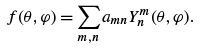If f(θ , ϕ ) is known, the coefﬁcients can be immediately found by the use of the orthogonality integral.

Table 12.4 Gravity Field Coefﬁcients, Eq. (12.156)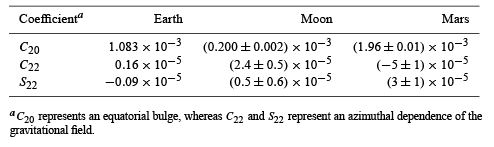Example : LAPLACE SERIES —GRAVITY FIELDS

The gravity ﬁelds of the Earth, the Moon, and Mars have been described by a Laplace series with real eigenfunctions: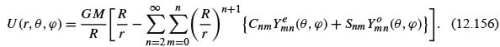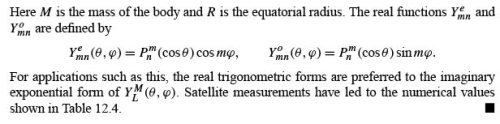ORBITAL ANGULAR MOMENTUM OPERATORS

Now we return to the speciﬁc orbital angular momentum operators Lx ,Ly , and Lz of quantum mechanics introduced in Section 4.3. Equation (4.68) becomes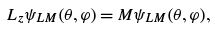and we want to show that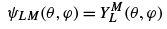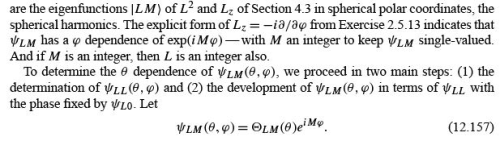From L+ ψLL = 0,L being the largest M , using the form of Lgiven in Exercises 2.5.14 and 12.6.7, we have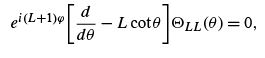(12.158)

and thus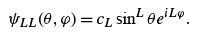(12.159)

Normalizing, we obtain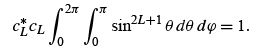(12.160)

The θ integral may be evaluated as a beta function (Exercise 8.4.9) and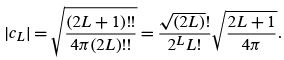(12.161)

This completes our ﬁrst step.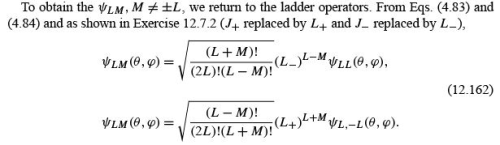Again, note that the relative phases are set by the ladder operators. Land L operating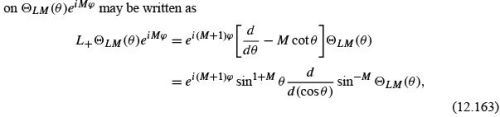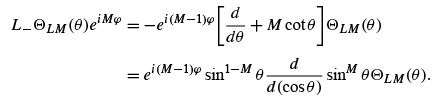Repeating these operations n times yields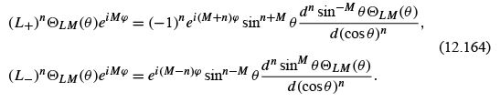From Eq. (12.163),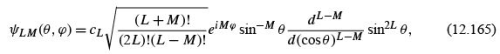and for M =−L: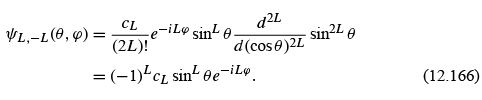Note the characteristic (−1)L phase of ψL,−L relative to ψL,L .This (−1)L enters from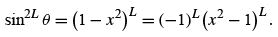(12.167)

Combining Eqs. (12.163), (12.163), and (12.166), we obtain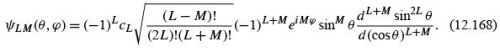Equations (12.165) and (12.168) agree if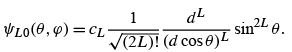(12.169)

Using Rodrigues’ formula, Eq. (12.65), we have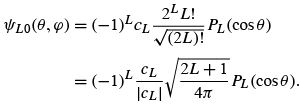(12.170)

The last equality follows from Eq. (12.161). We now demand that ψL0 (0, 0) be real and positive. Therefore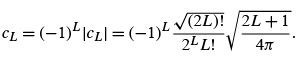(12.171)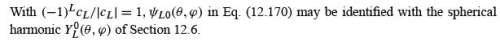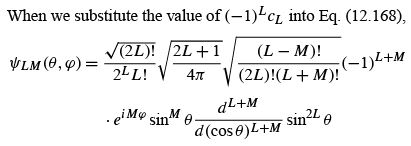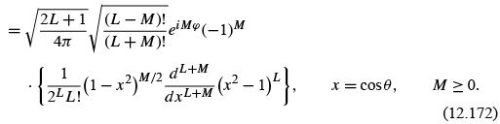The expression in the curly bracket is identiﬁed as the associated Legendre function (Eq. (12.151), and we have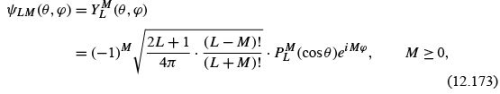in complete agreement with Section 12.6. Then by Eq. (12.73c),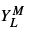for negative superscript is given by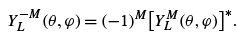(12.174)

• Our angular momentum eigenfunctions ψLM (θ , ϕ ) are identiﬁed with the spherical harmonics. The phase factor (−1)M is associated with the positive values of M and is seen to be a consequence of the ladder operators.
• Our development of spherical harmonics here may be considered a portion of Lie algebra.

THE ADDITION THEOREM FOR SPHERICAL HARMONICS

Trigonometric Identity

In the following discussion, (θ11 ) and (θ22 ) denote two different directions in our spherical coordinate system (x1 ,y1 ,z1 ), separated by an angle γ (Fig. 12.16). The polar angles θ1are measured from the z1 -axis. These angles satisfy the trigonometric identity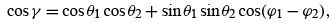(12.175)

which is perhaps most easily proved by vector methods
The addition theorem, then, asserts that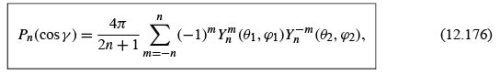or equivalently,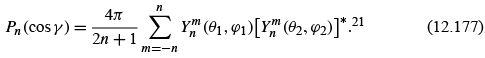In terms of the associated Legendre functions, the addition theorem is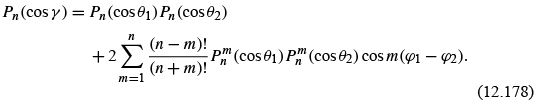Equation (12.175) is a special case of Eq. (12.178), n = 1.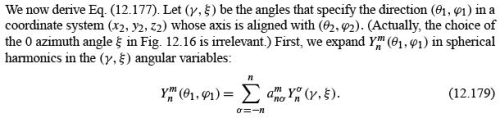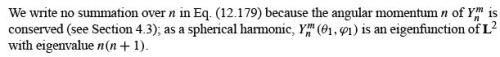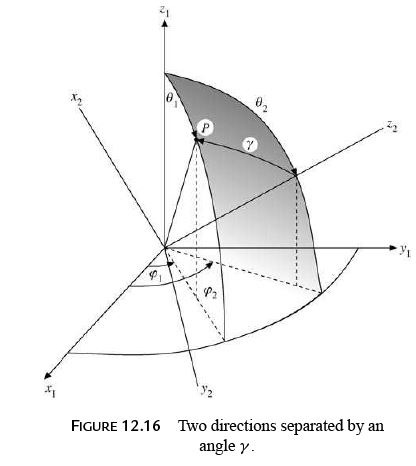We need for our proof only the coefﬁcient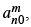which we get by multiplying Eq. (12.179) by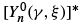and integrating over the sphere: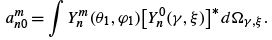(12.180)

Similarly, we expand P(cos γ) in terms of spherical harmonics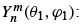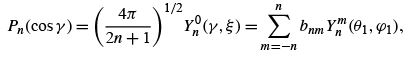(12.181)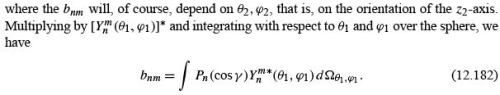In terms of spherical harmonics Eq. (12.182) becomes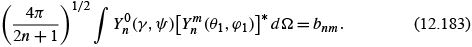Note that the subscripts have been dropped from the solid angle element dΩ Since the range of integration is over all solid angles, the choice of polar axis is irrelevant. Then comparing Eqs. (12.180) and (12.183), we see that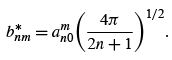(12.184)

Now we evaluate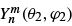using the expansion of Eq. (12.179) and noting that the values of (γ , ξ ) corresponding to (θ1) = (θ22 ) are (0, 0). The result is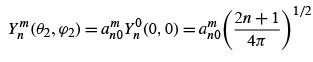(12.185)

all terms with nonzero σ vanishing. Substituting this back into Eq. (12.184), we obtain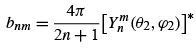(12.186)

Finally, substituting this expression for bnm into the summation, Eq. (12.181) yields Eq. (12.177), thus proving our addition theorem.
Those familiar with group theory will ﬁnd a much more elegant proof of Eq. (12.177) by using the rotation group.
One application of the addition theorem is in the construction of a Green’s function for the three-dimensional Laplace equation in spherical polar coordinates. If the source is on

the polar axis at the point (r = a, θ = 0,ϕ = 0), then, by Eq. (12.4a),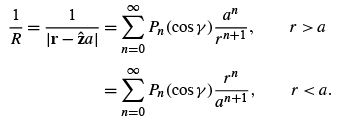(12.187)

Rotating our coordinate system to put the source at (a , θ22) and the point of observation at (r, θ11), we obtain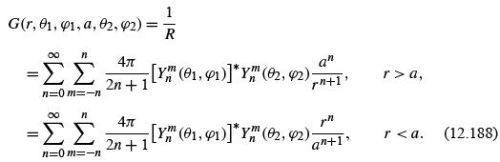Exercises

1. Two protons are uniformly distributed within the same spherical volume. If the coordinates of one element of charge are (r111 ) and the coordinates of the other are (r222 ) and r12 is the distance between them, the element of energy of repulsion will be given by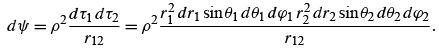Here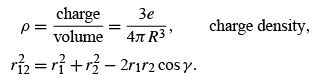Calculate the total electrostatic energy (of repulsion) of the two protons. This calculation is used in accounting for the mass difference in “mirror” nuclei, such as O 15 and N15 .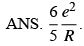This is double that required to create a uniformly charged sphere because we have two separate cloud charges interacting, not one charge interacting with itself (with permutation of pairs not considered).

2. Each of the two 1S electrons in helium may be described by a hydrogenic wave function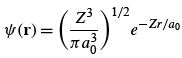in the absence of the other electron. Here Z , the atomic number, is 2. The symbol a0 is bthye Bohr radius, h¯ 2 /me2 . Find the mutual potential energy of the two electrons, given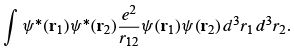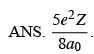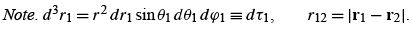3.  The probability of ﬁnding a 1 S hydrogen electron in a volume element r 2 dr sin θd θd ϕ is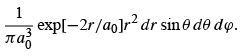Find the corresponding electrostatic potential. Calculate the potential from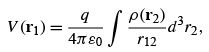with r1 not on the z-axis. Expand r12 . Apply the Legendre polynomial addition theorem and show that the angular dependence of V(r1 ) drops out.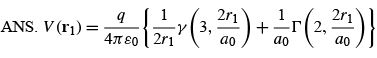Offer running on EduRev: Apply code STAYHOME200 to get INR 200 off on our premium plan EduRev Infinity!

159 docs

,

,

,

,

,

,

,

,

,

,

,

,

,

,

,

,

,

,

,

,

,

,

,

,

;